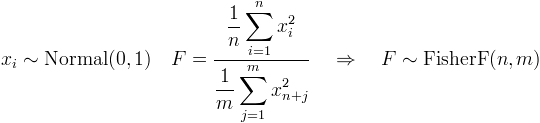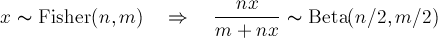﻿FisherDistribution Class# FisherDistribution Class

Represents the distribution of Fisher's F-statistic.Inheritance Hierarchy

Namespace:  Meta.Numerics.Statistics.Distributions
Assembly:  Meta.Numerics (in Meta.Numerics.dll) Version: 4.1.4Syntax
`public sealed class FisherDistribution : ContinuousDistribution`

The FisherDistribution type exposes the following members.Constructors
NameDescriptionFisherDistribution
Initializes a new Fisher distribution.
TopProperties
NameDescriptionDenominatorDegreesOfFreedom
Gets the number of degrees of freedom in the denominator.ExcessKurtosis
Gets the excess kurtosis of the distribution.
(Inherited from UnivariateDistribution.)Mean
Gets the mean of the distribution.
(Overrides UnivariateDistributionMean.)Median
Gets the median of the distribution.
(Inherited from ContinuousDistribution.)NumeratorDegreesOfFreedom
Gets the number of degrees of freedom in the numerator.Skewness
Gets the skewness of the distribution.
(Inherited from UnivariateDistribution.)StandardDeviation
Gets the standard deviation of the distribution.
(Inherited from UnivariateDistribution.)Support
Gets the interval over which the distribution is non-vanishing.
(Overrides ContinuousDistributionSupport.)Variance
Gets the variance of the distribution.
(Overrides UnivariateDistributionVariance.)
TopMethods
NameDescriptionCentralMoment
Computes a central moment of the distribution.
(Overrides ContinuousDistributionCentralMoment(Int32).)Cumulant
Computes a cumulant of the distribution.
(Inherited from UnivariateDistribution.)Equals
Determines whether the specified object is equal to the current object.
(Inherited from Object.)ExpectationValue
Computes the expectation value of the given function.
(Inherited from ContinuousDistribution.)GetHashCode
Serves as the default hash function.
(Inherited from Object.)GetRandomValue
Generates a random variate.
(Inherited from ContinuousDistribution.)GetRandomValues
Generates the given number of random variates.
(Inherited from ContinuousDistribution.)GetType
Gets the Type of the current instance.
(Inherited from Object.)Hazard
Computes the hazard function.
(Inherited from ContinuousDistribution.)InverseLeftProbability
Returns the point at which the cumulative distribution function attains a given value.
(Overrides ContinuousDistributionInverseLeftProbability(Double).)InverseRightProbability
Returns the point at which the right probability function attains the given value.
(Overrides ContinuousDistributionInverseRightProbability(Double).)LeftProbability
Returns the cumulative probability to the left of (below) the given point.
(Overrides ContinuousDistributionLeftProbability(Double).)ProbabilityDensity
Returns the probability density at the given point.
(Overrides ContinuousDistributionProbabilityDensity(Double).)RawMoment
Computes a raw moment of the distribution.
(Overrides ContinuousDistributionRawMoment(Int32).)RightProbability
Returns the cumulative probability to the right of (above) the given point.
(Overrides ContinuousDistributionRightProbability(Double).)ToString
Returns a string that represents the current object.
(Inherited from Object.)
TopRemarks

The ratio of the variances of two sets of normally distributed variables is distributed according to Fisher's F-distribution.Many test statistics are ratios of variances and are therefore distributed according to the F-distribution. These include the F-test (FisherFTest(Sample, Sample)), the goodness-of-fit test for a multi-linear regression (LinearRegression(Int32)), and ANOVA tests (OneWayAnovaTest(IReadOnlyCollectionSample)).

The Fisher distribution is related to the Beta distribution (BetaDistribution) by a simple variable transformation.See Also